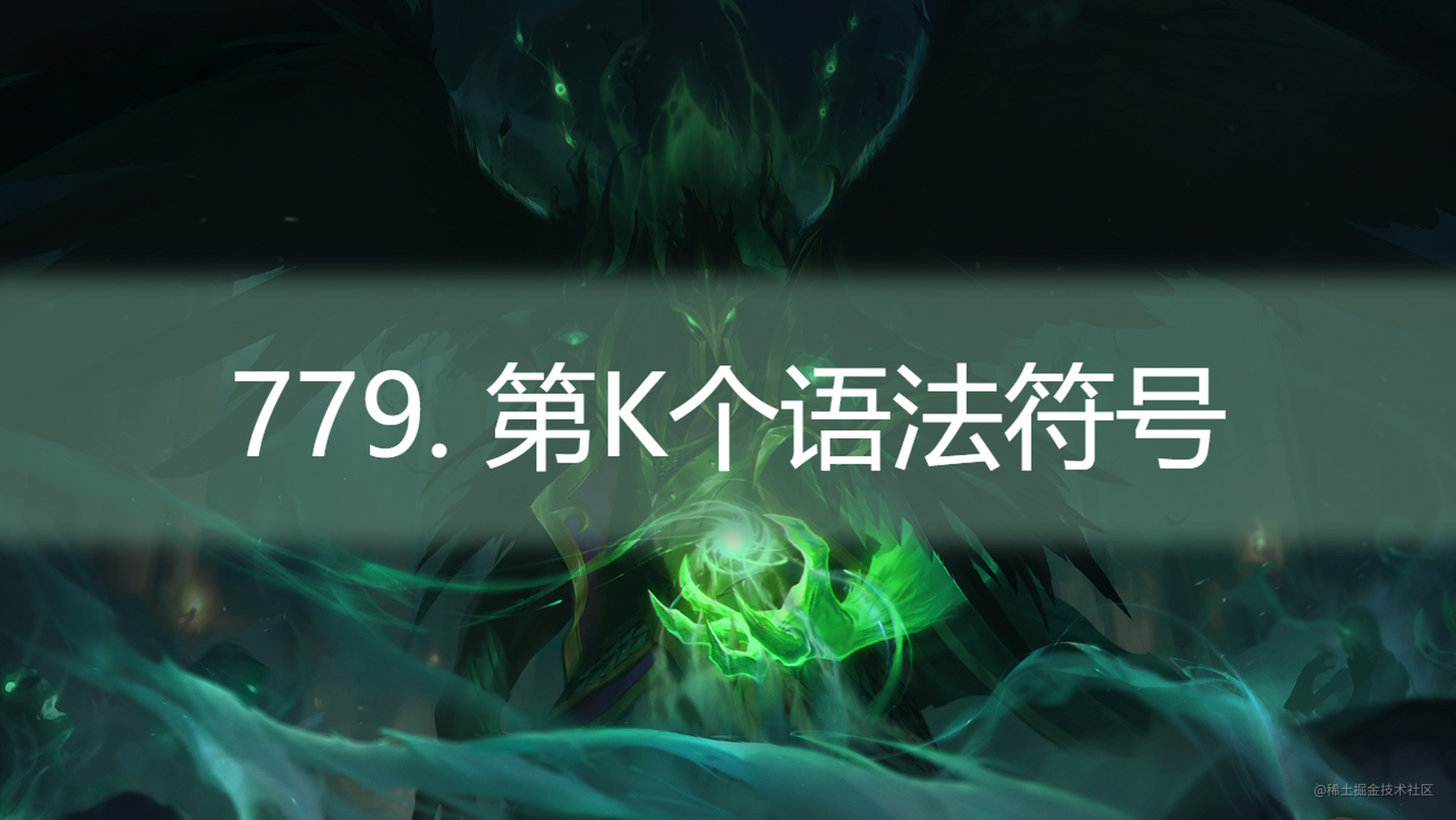# [路飞]_程序员必刷力扣题: 779. 第K个语法符号### 779. 第K个语法符号

``````输入: N = 1, K = 1

1. `N` 的范围 `[1, 30]`.
2. `K` 的范围 `[1, 2^(N-1)]`.

### 获取操作栈

• 暴力求解
• 按照题目要要求，生成每一行的字符串，然后直接获取第k个字符

• 获取操作栈

......

``````var kthGrammar = function (n, k) {
if (n === 1 && k === 1) return '0'
var treeStack = []
while (n > 1) {
var pos = k % 2 === 0 ? 1 : 0
var opt = [n, k, pos]
k = Math.ceil(k / 2)
treeStack.push(opt)
n--
}
var curr = '0'
while (treeStack.length) {
var item = treeStack.pop()
var newCurr = curr === '1' ? '10' : '01'
var index = item
curr = newCurr[index]
}
return curr
};